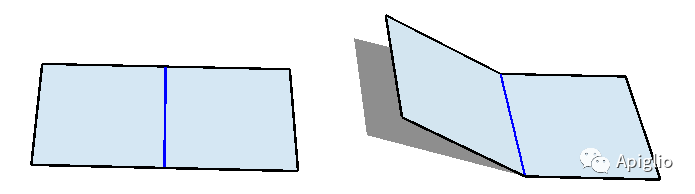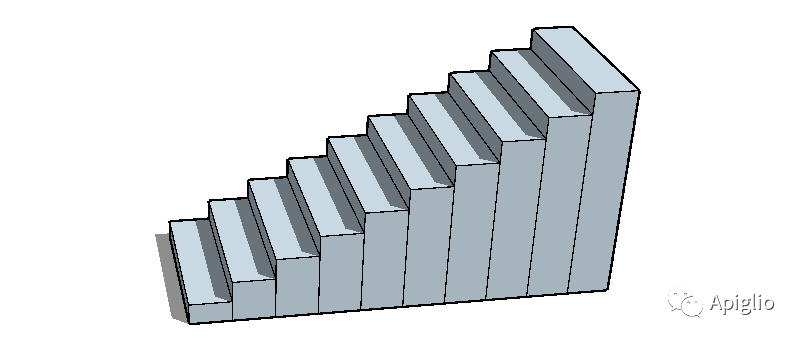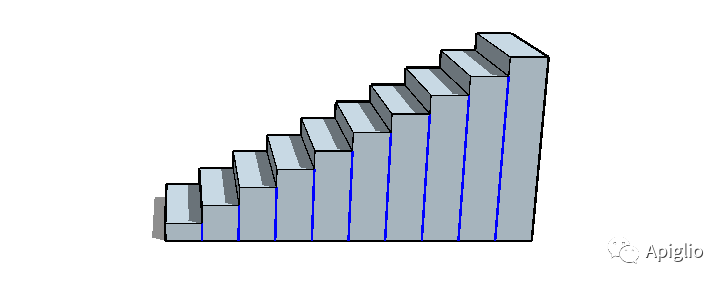# 【零基础】Sketchup删除平面内边线

· 2021-09-17

础  本系列将回顾和串联往期几篇文章中的部分内容，并通过具体的例子作尽可能详尽的操作介绍。其中的部分细节和特殊情况将不再重复描述，专注于 零基础  step-by-step 两个属性。

 【本期导览】 〇、何谓“平面内边线”一、原理介绍 二、代码实现三、补充说明

〇、何谓“平面内边线”①首先要是边线图元（Sketchup:: Edge）；

②连接且只连接 2 个平面图元（Sketchup:: Face）；

③所连接的两个平面图元相互平行。

``  if edg.is_a?(Sketchup::Edge) then``    if edg.faces.length !=2 then``      false``    else``      edg.faces.normal.parallel?(edg.faces.normal)``    end``  else``    false``  end``

``sels=Sketchup.active_model.selection.to_a``sels.select!{|edg|``  if edg.is_a?(Sketchup::Edge) then``    if edg.faces.length !=2 then``      false``    else``      edg.faces.normal.parallel?(edg.faces.normal)``    end``  else``    false``  end``}``Sketchup.active_model.selection.clear``Sketchup.active_model.selection.add(sels)````def inner_edge``  #在这里复制上文的代码实现``end``

``module Sel``  def Sel.inner``    Sel.reselect{|edg|``      if edg.is_a?(Sketchup::Edge) then``        if edg.faces.length !=2 then``          false``        else``          edg.faces.normal.parallel?(edg.faces.normal)``        end``      else``        false``      end``    }``  end``end``

（完）